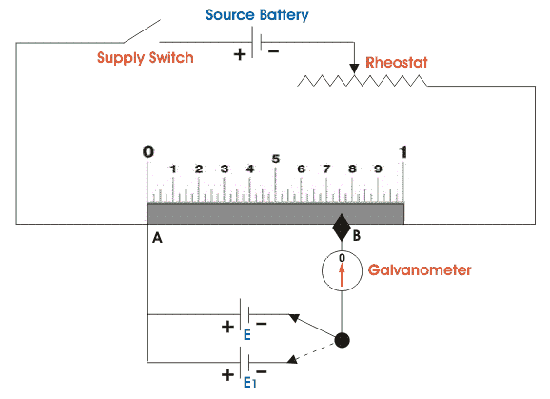# Current electricity

QuestionsCategory: High School PhysicsCurrent electricity

When 6 identical cells of no resistance are connected in series in the secondary circuit,of a potentiometer,the balancing length is l,balance length becomes l/3 when some cells are connected wrongly then the no of cells connected wrongly are?

I am not really sure but I guess, 2 cells are wrongly connected. Do you have the answer to this question?

Yes but How

It’s been a long time since I read about potentiometers, or tried any numerical on them, so I might be wrong.
But since the answer that I got is right, let me explain how I arrived at that.The above picture shows a potentiometer, where E is the emf of the standard cell.
And E1 is the emf of the additional cells that you are going to provide.
Now the important relation between E and E1 is given as:$\frac{E_1}{E}=\frac{L_1}{L}$
So, you say that when we connect 6 cells, then the balancing length, i.e.$L_1=l$
Which means,$\frac{E_1}{E}=\frac{l}{L} .........(1)$
But, when we change the arrangement of cells, then the balancing length,$L_1'=\frac{l}{3}$$\implies \frac{E_1'}{E}=\frac{l}{3L}........(2)$

Dividing (1) by (2) we get,$\frac{E_1}{E_1'}={3}$

So, how can the new arrangement of cells have an EMF which is one third times of that when the cells are in series.
It can happen if 4 cells are connected in series and 2 of them are wrongly connected.
Let’s say each cell has an EMF of 1 volts.
Then$E_1'=4V-2V=2$ Volts(when 2 cells are wrongly connected).
and$E_1=6V$ (cells in series)

Therefore,$\frac{E_1}{E_1'}=3$
Which is what we got earlier using the balance length formula.

NOTE: This forum is for conceptual questions. Next time you ask such a question, you should at least tell us what you tried and what approach you are using.
Then we will try to point you in the right direction.
The site isn’t for solving your homework.

Hope you find it useful!

So, how can the new arrangement of cells have an EMF which is one third times of that when the cells are in series.
It can happen if 4 cells are connected in series and 2 of them are wrongly connected.

I didn’t understand this part

And this is not my homework,I am preparing for olympiads

E1′ is the emf of the new arrangement where some cells maybe wrongly connected. E1’/E1=1/3(calculated).If each cell has the potential of 1 V, then when they are all in series(the initial arrangement) then E1=6V.
If in the new arrangement we connect 4 in series but 2 in the opp. direction, then E1’=4-2=2V.
So, E1’/ E1=1/3.
Give it some thought. I don’t know any better way to explain it.

Got it